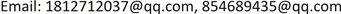﻿ 基于灰色关联度和协方差的学生成绩实证分析 An Empirical Analysis of Students’ Achievement Based on Grey Correlation Degree and Covariance

Statistics and Application
Vol. 07  No. 05 ( 2018 ), Article ID: 27179 , 6 pages
10.12677/SA.2018.75056

An Empirical Analysis of Students’ Achievement Based on Grey Correlation Degree and Covariance

Yi Liu, Heling Wang

Xinjiang University of Finance and Economics, Urumqi XinjiangReceived: Sep. 30th, 2018; accepted: Oct. 12th, 2018; published: Oct. 19th, 2018ABSTRACT

In this paper, the mathematics scores of nine majors students in Xinjiang University of Finance and Economics from 2003 to 2016 and the scores of linear algebra, calculus, probability theory of 10 semesters in the undergraduate period are selected to sort out and statistics and the data are sorted out with the help of MATLAB, SPSS. This paper discusses the grey correlation degree between the mathematics achievement of college entrance examination and the achievement of some representative mathematics courses in university, and finds that some representative mathematics courses have a high correlation degree with the mathematics achievement of college entrance examination. Then, by means of ANOVA and covariance analysis, the author discusses the factors that affect the achievement of college mathematics, and draws the conclusion that the teaching level of the teachers in the course is very important. There is no significant difference in the analysis of college mathematics scores, but the college entrance examination results have a significant impact on the university mathematics scores. On this basis, we analyze the reasons for its formation and give some suggestions on the characteristics of college mathematics learning.

Keywords:Grey Correlation Analysis, Analysis of Variance, Covariance Analysis1. 引言

2. 研究方法及研究对象

2.1. 研究方法

2.2. 研究对象及数据处理

3. 基于MATLAB的灰色关联度分析

3.1. 选择参考数列与比较数列

3.2. 对各数列进行无量纲化处理

3.3. 计算参考数列与比较数列之间的灰色关联系数

3.4. 计算关联度

3.5. 关联度的排序

4. 基于SPSS的协方差分析

4.1. 协方差统计原理

${Q}_{总}={Q}_{控制变量}+{Q}_{协变量}+{Q}_{随机因素}$

${F}_{控制变量}=\frac{{S}_{控制变量}^{2}}{{S}_{随机因素}^{2}},\text{\hspace{0.17em}}{F}_{协变量}=\frac{{S}_{协变量}^{2}}{{S}_{随机因素}^{2}}$

4.2. 协方差分析步骤Table 2. Difference dependent test variables: linear algebra scores

5. 结论与建议

5.1. 注重数学教育观念的培养Table 3. Interaction test between covariate and dependent variable

5.2. 突出数学的实用性和专业性

5.3. 数学课程与其他课程的结合

An Empirical Analysis of Students’ Achievement Based on Grey Correlation Degree and Covariance[J]. 统计学与应用, 2018, 07(05): 483-488. https://doi.org/10.12677/SA.2018.75056

1. 1. 郑楚云. 数学成绩与专业课成绩间的影响分析[D]: [硕士学位论文]. 桂林: 广西师范大学, 2013.

2. 2. 吴艳萍, 郑维, 孙菲, 张璐寒, 徐义敏. 大学生数学成绩影响因素的灰色关联分析[J]. 数学学习与研究, 2017(15): 4-5.

3. 3. 汪朝杰, 谭常春, 汪慧. 大学生在校成绩与高考成绩的统计分析[J]. 大学数学, 2013, 29(4): 79-86.

4. 4. 刘利明, 蔺小林, 桑园. 学生成绩的可控影响因子分析——以高等数学为例[J]. 中国科教创新导刊, 2009(19): 143.

5. 5. 闫晓毓. 对普通高校体育教师评价指标体系的研究[D]: [硕士学位论文]. 成都: 西南交通大学, 2017.

6. 6. 何阅雄, 李茂森, 高鸾. 教师发展视域下的教师评价机制的思考与实践[J]. 高等工程教育研究, 2016(1): 107-112.